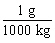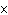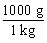Name:    Dimensional Analysis/Conversions Quiz

Multiple Choice
Identify the choice that best completes the statement or answers the question.

1.

A conversion factor that shows the relationship between grams and kilograms is
 a.b.1000 c.d. ÷ 1000

2.

What is the temperature of absolute zero (0 K) measured inC?
 a. –73C b. –373C c. –173C d. –273C

3.

Which temperature scale has no negative temperatures?
 a. Celsius b. Joule c. Fahrenheit d. Kelvin

4.

What is the temperature –34C expressed in kelvins?
 a. 139 K b. 239 K c. 339 K d. 207 K

5.

If the temperature changes by 100 K, by how much does it change inC?
 a. 100C b. 0C c. 37C d. 273C

6.

Chlorine boils at 239 K. What is the boiling point of chlorine expressed in degrees Celsius?
 a. –34C b. 93C c. –61C d. 34C

7.

A train travels at a speed of 30 miles per hour. If 1 mile = 1.6 kilometers, how fast is the train traveling in kilometers per minute?
 a. 0.8 km/min b. 0.4 km/min c. 0.6 km/min d. 1.0 km/min

8.

Jennifer downloaded a 1,000,000-byte file in 200 seconds. At the same rate, how long will it take Jennifer to download a 3,500,000-byte file?
 a. 500 s b. 900 s c. 350 s d. 600 s e. 700 s

9.

Maurice is filling a 10-gallon aquarium with water at the rate of 1 quart every 3 minutes. How many hours will it take Maurice to fill the aquarium? (1 gallon = 4 quarts,  60 min = 1 hou)
 a. 1.2 h b. 12 h c. 0.4 h d. 2 h e. 20 h

10.

Which of the following conversion factors would you use to change 18 kilometers to meters?
 a. 1000 m/1 km c. 100 m/1 km b. 1 km/1000 m d. 1 km/100 m